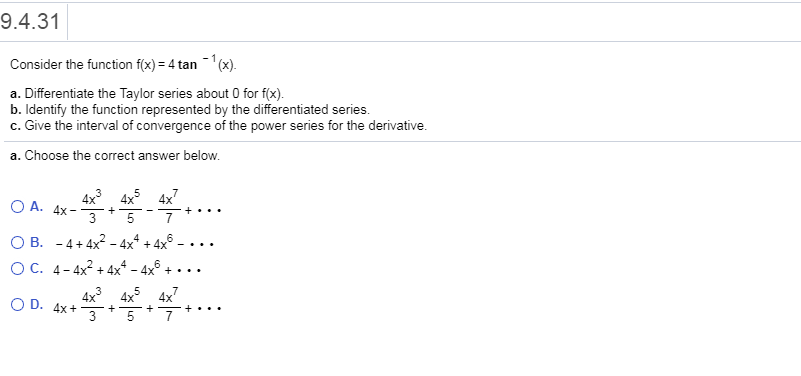# 9.4.31 Consider the function fx)-4 tan (x) a. Differentiate the Taylor series about 0 for f(x). b. Identify the function represented by the differentiated series. c. Give the interval of convergence of the power series for the derivative a. Choose the correct answer below. 4x 4x 4x OB. -4+4x2 _ 4x4 + 4x6-... O C. 4-4x+4x4-4x6+... O D. 4x+... 4x 4x 4x

Question

9.4.31help_outlineImage Transcriptionclose9.4.31 Consider the function fx)-4 tan (x) a. Differentiate the Taylor series about 0 for f(x). b. Identify the function represented by the differentiated series. c. Give the interval of convergence of the power series for the derivative a. Choose the correct answer below. 4x 4x 4x OB. -4+4x2 _ 4x4 + 4x6-... O C. 4-4x+4x4-4x6+... O D. 4x+... 4x 4x 4x fullscreen